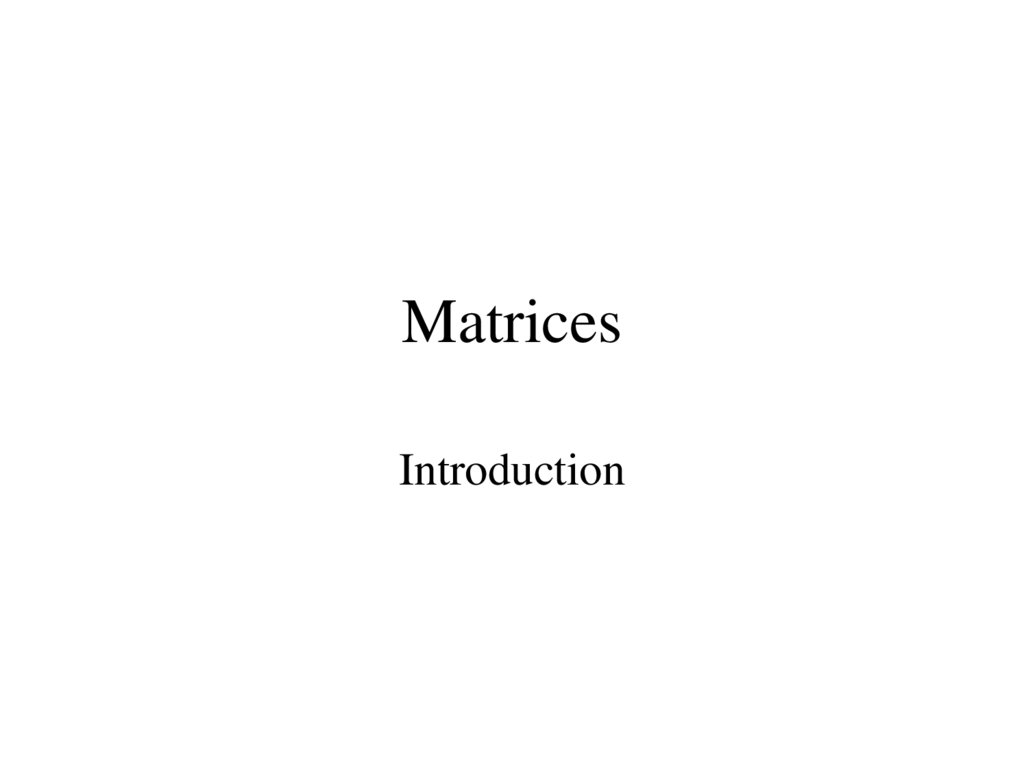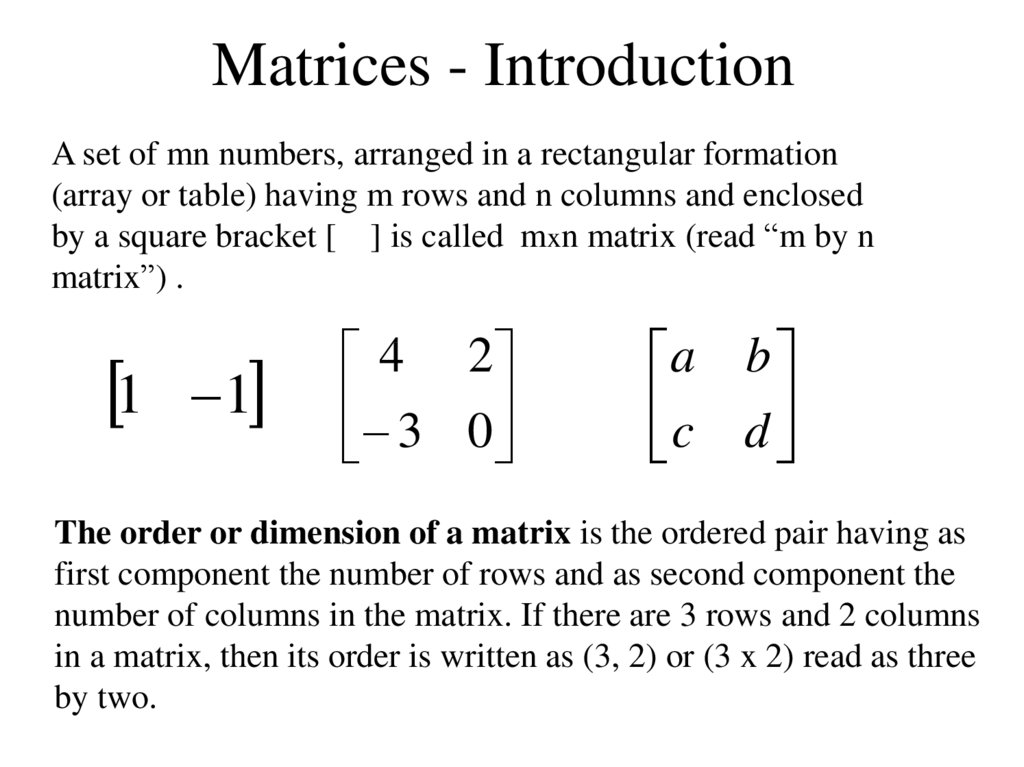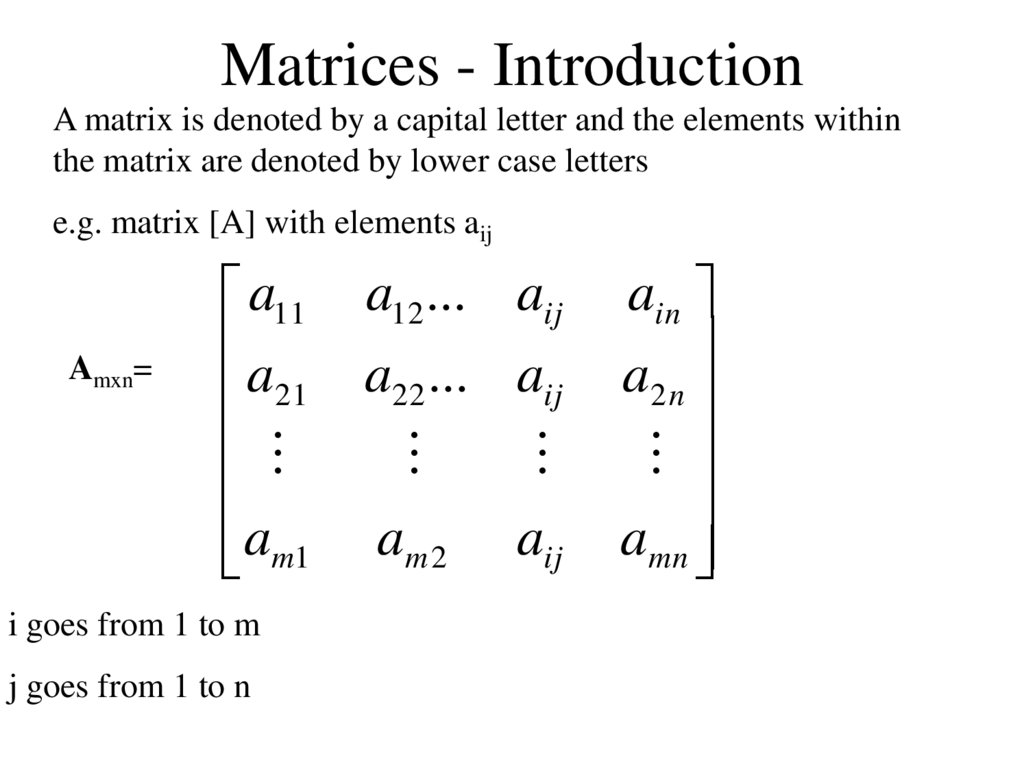# Matrices - Introduction. Lecture 1-3

Introduction

## 2. Matrices - Introduction

A set of mn numbers, arranged in a rectangular formation
(array or table) having m rows and n columns and enclosed
by a square bracket [ ] is called mxn matrix (read “m by n
matrix”) .
1
1
4 2
3 0
a b
c d
The order or dimension of a matrix is the ordered pair having as
first component the number of rows and as second component the
number of columns in the matrix. If there are 3 rows and 2 columns
in a matrix, then its order is written as (3, 2) or (3 x 2) read as three
by two.

## 3. Matrices - Introduction

A matrix is denoted by a capital letter and the elements within
the matrix are denoted by lower case letters
e.g. matrix [A] with elements aij
Amxn=
a11
a
21
am1
i goes from 1 to m
j goes from 1 to n
a12 ... aij
a22 ... aij
am 2
aij
ain
a2 n
amn

## 4. Matrices - Introduction

TYPES OF MATRICES
1. Column matrix or vector:
The number of rows may be any integer but the number of
columns is always 1
1
4
2
1
3
a11
a21
am1

## 5. Matrices - Introduction

TYPES OF MATRICES
2. Row matrix or vector
Any number of columns but only one row
1
1 6
a11
a12
0
3 5 2
a13 a1n

## 6. Matrices - Introduction

TYPES OF MATRICES
3. Rectangular matrix
Contains more than one element and number of rows is not
equal to the number of columns
1 1
3 7
7 7
7 6
1 1 1 0 0
2 0 3 3 0
m n

## 7. Matrices - Introduction

TYPES OF MATRICES
4. Square matrix
The number of rows is equal to the number of columns
(a square matrix A has an order of m)
mxm
1 1
3 0
1 1 1
9 9 0
6 6 1
The principal or main diagonal of a square matrix is composed of all
elements aij for which i=j

## 8. Matrices - Introduction

TYPES OF MATRICES
5. Diagonal matrix
A square matrix where all the elements are zero except those on
the main diagonal
1 0 0
0 2 0
0 0 1
i.e. aij =0 for all i = j
aij = 0 for some or all i = j
3
0
0
0
0 0 0
3 0 0
0 5 0
0 0 9

## 9. Matrices - Introduction

TYPES OF MATRICES
6. Unit or Identity matrix - I
A diagonal matrix with ones on the main diagonal
1
0
0
0
0 0 0
1 0 0
0 1 0
0 0 1
i.e. aij =0 for all i = j
aij = 1 for some or all i = j
1 0
0 1
aij
0
0
aij

## 10. Matrices - Introduction

TYPES OF MATRICES
7. Null (zero) matrix - 0
All elements in the matrix are zero
0
0
0
aij 0
0 0 0
0 0 0
0 0 0
For all i,j

## 11. Matrices - Introduction

TYPES OF MATRICES
8. Triangular matrix
A square matrix whose elements above or below the main
diagonal are all zero
1 0 0
2 1 0
5 2 3
1 0 0
2 1 0
5 2 3
1 8 9
0 1 6
0 0 3

## 12. Matrices - Introduction

TYPES OF MATRICES
8a. Upper triangular matrix
A square matrix whose elements below the main
diagonal are all zero
aij
0
0
aij
aij
0
aij
aij
aij
i.e. aij = 0 for all i > j
1 8 7
0 1 8
0 0 3
1
0
0
0
7 4 4
1 7 4
0 7 8
0 0 3

## 13. Matrices - Introduction

TYPES OF MATRICES
8b. Lower triangular matrix
A square matrix whose elements above the main diagonal are all
zero
aij
aij
aij
0
aij
aij
0
0
aij
i.e. aij = 0 for all i < j
1 0 0
2 1 0
5 2 3

## 14. Matrices – Introduction

TYPES OF MATRICES
9. Scalar matrix
A diagonal matrix whose main diagonal elements are
equal to the same scalar
A scalar is defined as a single number or constant
aij
0
0
0
aij
0
0
0
aij
i.e. aij = 0 for all i = j
aij = a for all i = j
1 0 0
0 1 0
0 0 1
6
0
0
0
0 0 0
6 0 0
0 6 0
0 0 6

## 15. Matrices

Matrix Operations

## 16. Matrices - Operations

EQUALITY OF MATRICES
Two matrices are said to be equal only when all
corresponding elements are equal
Therefore their size or dimensions are equal as well
A=
1 0 0
2 1 0
5 2 3
B=
1 0 0
2 1 0
5 2 3
A=B

## 17. Matrices - Operations

Some properties of equality:
•IIf A = B, then B = A for all A and B
•IIf A = B, and B = C, then A = C for all A, B and C
A=
1 0 0
2 1 0
5 2 3
If A = B then
aij bij
b11 b12 b13
B=
b
b
b
21
22
23
b31 b32 b33

## 18. Matrices - Operations

The sum or difference of two matrices, A and B of the same
size yields a matrix C of the same size
cij aij bij
Matrices of different sizes cannot be added or subtracted

## 19. Matrices - Operations

Commutative Law:
A+B=B+A
Associative Law:
A + (B + C) = (A + B) + C = A + B + C
5 6 8
8 5
7 3 1 1
2 5 6 4 2 3 2 7 9
A
2x3
B
2x3
C
2x3

## 20. Matrices - Operations

A+0=0+A=A
A + (-A) = 0 (where –A is the matrix composed of –aij as elements)
6 4 2 1 2 0 5 2 2
3 2 7 1 0 8 2 2 1

## 21. Matrices - Operations

SCALAR MULTIPLICATION OF MATRICES
Matrices can be multiplied by a scalar (constant or single
element)
Let k be a scalar quantity; then
kA = Ak
Ex. If k=4 and
3 1
2 1
A
2 3
4 1

## 22. Matrices - Operations

3 1 3 1
12 4
2 1 2 1
8 4
4
4
2 3 2 3
8 12
4 1 4 1
16 4
Properties:
• k (A + B) = kA + kB
• (k + g)A = kA + gA
• k(AB) = (kA)B = A(k)B
• k(gA) = (kg)A

## 23. Matrices - Operations

MULTIPLICATION OF MATRICES
The product of two matrices is another matrix
Two matrices A and B must be conformable for multiplication to
be possible
i.e. the number of columns of A must equal the number of rows
of B
Example.
A
(1x3)
x
B =
(3x1)
C
(1x1)

B x
A
=
Not possible!
(2x1) (4x2)
A
x
B
=
Not possible!
(6x2) (6x3)
Example
A
(2x3)
x
B
(3x2)
=
C
(2x2)

## 25. Matrices - Operations

a11 a12
a
21 a22
b11 b12
a13
c11 c12
b
b
21
22
a23
c21 c22
b31 b32
(a11 b11 ) (a12 b21 ) (a13 b31 ) c11
(a11 b12 ) (a12 b22 ) (a13 b32 ) c12
(a21 b11 ) (a22 b21 ) (a23 b31 ) c21
(a21 b12 ) (a22 b22 ) (a23 b32 ) c22
Successive multiplication of row i of A with column j of
B – row by column multiplication

## 26. Matrices - Operations

4 8
1 2 3
(1 4) (2 6) (3 5) (1 8) (2 2) (3 3)
4 2 7 6 2 (4 4) (2 6) (7 5) (4 8) (2 2) (7 3)
5 3
31 21
63 57
Remember also:
IA = A
1 0 31 21
0 1 63 57
31 21
63 57

## 27. Matrices - Operations

Assuming that matrices A, B and C are conformable for
the operations indicated, the following are true:
1. AI = IA = A
2. A(BC) = (AB)C = ABC -
(associative law)
3. A(B+C) = AB + AC - (first distributive law)
4. (A+B)C = AC + BC - (second distributive law)
Caution!
1. AB not generally equal to BA, BA may not be conformable
2. If AB = 0, neither A nor B necessarily = 0
3. If AB = AC, B not necessarily = C

## 28. Matrices - Operations

AB not generally equal to BA, BA may not be conformable
1 2
T
5
0
3 4
S
0
2
1 2 3
TS
5 0 0
3 4 1
ST
0 2 5
4 3
2 15
2 23
0 10
8
20
6
0

## 29. Matrices - Operations

If AB = 0, neither A nor B necessarily = 0
3 0 0
1 1 2
0 0 2 3 0 0

## 30. Matrices - Operations

TRANSPOSE OF A MATRIX
If :
2 4 7
A 2 A
2x3
5 3 1
3
Then transpose of A, denoted AT is:
3T
A 2 A
T
2 5
4 3
7 1
aij a
T
ji
For all i and j

## 31. Matrices - Operations

To transpose:
Interchange rows and columns
The dimensions of AT are the reverse of the dimensions of A
2 4 7
A 2 A
5
3
1
3
T2
A 3 A
T
2 5
4 3
7 1
2x3
3x2

## 32. Matrices - Operations

Properties of transposed matrices:
1. (A+B)T = AT + BT
2. (AB)T = BT AT
3. (kA)T = kAT
4. (AT)T = A

## 33. Matrices - Operations

1. (A+B)T = AT + BT
5 6 8
8 5
7 3 1 1
2 5 6 4 2 3 2 7 9
2 1 4 8 2
7
3 5 5 2 8 7
1 6 6 3 5 9
8 2
8 7
5 9

(AB)T = BT AT
1
1 1 0 2
0 2 3 1 8 2 8
2
1 0
1 1 2 1 2 2 8
0 3

## 35. Matrices - Operations

SYMMETRIC MATRICES
A Square matrix is symmetric if it is equal to its
transpose:
A = AT
a b
A
b d
a b
T
A
b
d

## 36. Matrices - Operations

When the original matrix is square, transposition does not
affect the elements of the main diagonal
a b
A
c
d
a c
T
A
b
d
The identity matrix, I, a diagonal matrix D, and a scalar matrix, K,
are equal to their transpose since the diagonal is unaffected.

## 37. Matrices - Operations

INVERSE OF A MATRIX
Consider a scalar k. The inverse is the reciprocal or division of 1
by the scalar.
Example:
k=7
the inverse of k or k-1 = 1/k = 1/7
Division of matrices is not defined since there may be AB = AC
while B = C
The inverse of a square matrix, A, if it exists, is the unique matrix
A-1 where:
AA-1 = A-1 A = I

Example:
3
A 2 A
2
1
1
A
2
2
Because:
1
1
1
3
1 1 3 1 1
2 3 2 1 0
3 1 1 1 1
2 1 2 3 0
0
1
0
1

## 39. Matrices - Operations

Properties of the inverse:
( AB) 1 B 1 A 1
1 1
(A ) A
T 1
1 T
(A ) (A )
1 1
1
(kA) A
k
A square matrix that has an inverse is called a nonsingular matrix
A matrix that does not have an inverse is called a singular matrix
Square matrices have inverses except when the determinant is zero
When the determinant of a matrix is zero the matrix is singular

## 40. Matrices - Operations

DETERMINANT OF A MATRIX
To compute the inverse of a matrix, the determinant is required
Each square matrix A has a unit scalar value called the
determinant of A, denoted by det A or |A|
If
then
1
A
6
1
A
6
2
5
2
5

## 41. Matrices - Operations

If A = [A] is a single element (1x1), then the determinant is
defined as the value of the element
Then |A| =det A = a11
If A is (n x n), its determinant may be defined in terms of order
(n-1) or less.

## 42. Matrices - Operations

MINORS
If A is an n x n matrix and one row and one column are deleted,
the resulting matrix is an (n-1) x (n-1) submatrix of A.
The determinant of such a submatrix is called a minor of A and
is designated by mij , where i and j correspond to the deleted
row and column, respectively.
mij is the minor of the element aij in A.

## 43. Matrices - Operations

eg.
a11 a12
A a21 a22
a31 a32
a13
a23
a33
Each element in A has a minor
Delete first row and column from A .
The determinant of the remaining 2 x 2 submatrix is the minor
of a11
m11
a22
a23
a32
a33

## 44. Matrices - Operations

Therefore the minor of a12 is:
m12
a21 a23
a31 a33
And the minor for a13 is:
m13
a21 a22
a31 a32

## 45. Matrices - Operations

COFACTORS
The cofactor Cij of an element aij is defined as:
Cij ( 1)i j mij
When the sum of a row number i and column j is even, cij = mij and
when i+j is odd, cij =-mij
c11 (i 1, j 1) ( 1)1 1 m11 m11
1 2
m12 m12
1 3
m13 m13
c12 (i 1, j 2) ( 1)
c13 (i 1, j 3) ( 1)

## 46. Matrices - Operations

DETERMINANTS CONTINUED
The determinant of an n x n matrix A can now be defined as
A det A a11c11 a12c12 a1nc1n
The determinant of A is therefore the sum of the products of the
elements of the first row of A and their corresponding cofactors.
(It is possible to define |A| in terms of any other row or column
but for simplicity, the first row only is used)

## 47. Matrices - Operations

Therefore the 2 x 2 matrix :
a11 a12
A
a21 a22
Has cofactors :
c11 m11 a22 a22
And:
c12 m12 a21 a21
And the determinant of A is:
A a11c11 a12c12 a11a22 a12a21

## 48. Matrices - Operations

Example 1:
3 1
A
1
2
A (3)(2) (1)(1) 5

## 49. Matrices - Operations

For a 3 x 3 matrix:
a11 a12
A a21 a22
a31 a32
a13
a23
a33
The cofactors of the first row are:
c11
a22
a23
a32
a33
a22a33 a23a32
a21 a23
c12
(a21a33 a23a31 )
a31 a33
a21 a22
c13
a21a32 a22a31
a31 a32

## 50. Matrices - Operations

The determinant of a matrix A is:
A a11c11 a12c12 a11a22 a12a21
Which by substituting for the cofactors in this case is:
A a11(a22a33 a23a32 ) a12 (a21a33 a23a31) a13 (a21a32 a22a31)

## 51. Matrices - Operations

Example 2:
1 0 1
A 0 2 3
1 0 1
A a11(a22a33 a23a32 ) a12 (a21a33 a23a31) a13 (a21a32 a22a31)
A (1)(2 0) (0)(0 3) (1)(0 2) 4

## 52. Matrices - Operations

A cofactor matrix C of a matrix A is the square matrix of the same
order as A in which each element aij is replaced by its cofactor cij .
Example:
If
1 2
A
3 4
The cofactor C of A is
4 3
C
2
1

## 53. Matrices - Operations

The adjoint matrix of A, denoted by adj A, is the transpose of its
cofactor matrix
T
It can be shown that:
Example:
1 2
A
3
4
A (1)( 4) (2)( 3) 10
4 2
3
1
T

1 2 4 2 10 0
10 I
3 4 3 1 0 10
4 2 1 2 10 0
10 I
3 1 3 4 0 10

## 55. Matrices - Operations

USING THE ADJOINT MATRIX IN MATRIX INVERSION
Since
AA-1 = A-1 A = I
and
then
A
A
1

Example
A=
1 2
3 4
1 4 2 0.4 0.2
A
10 3 1 0.3 0.1
1
To check
AA-1 = A-1 A = I
1 2 0 . 4 0 .2 1
AA
3 4 0.3 0 .1 0
0 .4 0 . 2 1 2 1
1
A A
0.3 0.1 3 4 0
1
0
I
1
0
I
1

## 57. Matrices - Operations

Example 2
3 1 1
A 2 1 0
1 2 1
The determinant of A is
|A| = (3)(-1-0)-(-1)(-2-0)+(1)(4-1) = -2
The elements of the cofactor matrix are
c11 ( 1),
c12 ( 2),
c13 (3),
c21 ( 1),
c22 ( 4),
c23 (7),
c31 ( 1),
c32 ( 2),
c33 (5),

## 58. Matrices - Operations

The cofactor matrix is therefore
3
1 2
C 1 4 7
1 2
5
so
and
1 1 1
adjA C T 2 4 2
3 7 5
1 1 1 0.5 0.5 0.5
1.0 2.0 1.0
1
A
2
4
2
A
2
3 7 5 1.5 3.5 2.5

## 59. Matrices - Operations

The result can be checked using
AA-1 = A-1 A = I
The determinant of a matrix must not be zero for the inverse to
exist as there will not be a solution
Nonsingular matrices have non-zero determinants
Singular matrices have zero determinants